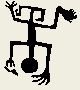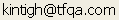Tools for Quantitative Archaeology    Email:RANDPT: Generate Random Point Distributions

RANDPT will generate random distributions of points using different randomization models. It will also random walk points in an existing distribution. The program is available as freeware in randpt.zip.

PROGRAM OPERATION

[G]enerate Random Points or Random [W]alk Existing Points {G} ?

Answer G to generate a set of random points or W to apply a random walk to a distribution of points.

To Generate Points

Distribution Shape: [C]ircular or [R]ectangular {R} ?

Indicates the shape of the limits of the distribution. Points will not eb generated outside the limits. More complex shapes are not allowed.

Distribution Diameter {20.0} ?

X Coord, Center {10.0} ?

Y Coord, Center {10.0} ?
or

Xmin {0.0} ?

Xmax {0.0} ?

Ymin ?

Ymax ?

Define the distribution limits by diamter and center or corner coordinates.

Random Generator Seed {0} ?

Seed the Random Number Generator.

[N]umber or [D]ensity of Random Points to be Specified {N} ?

The program will generate either a fixed number of points or calculate a number of points based on the density in points per square unit based on the site size.

Number of Points ?

[C]entered or Contagious Around [S]eeds

"Center" generated points in a single cluster centered on the distribution limits. "Contagious" establishes a number of random seeds around which points are clustered. Use contagious to produce a patchy distribution. Use Center for a simple random distribution.

Number of Seeds ?

Use Cluster Seeds as Points ?

Choose whether or not the cluster seeds will count as points. Otherwise they will be invisible.

Size of Contagious Radius or Stddev ?

A parameter that specifies the cluster “size” in the input units. For all but Normal (below) this specifies a radius. For Normal it specifies the number of units associated with 1 std.

Density Distribution Model

[U]niform [H]emisphere [C]onic [S]inusoid [N]ormal {N} ?

Centered distributions act like seeded distributions with a single seed at the center and a seed radius the same as distribution limit radius (circular distributions) or the half the width of the limit (rectangular distributions). For contagious distributions: a Uniform model provides for equal probability for all points within the radius; a Hemispheric model provides that the probabilities at a given distance from the seed will fall off from a maximum at the seed to 0 at the edge with intermediate distributions following a hemispheric model (relatively slow falloff from the center); a Conic distribution has probabilities fall off linearly from the center to the edge; a Siusoidal model follows a sine curve (a rapid falloff from the center); a Normal model has probabilities dictated by the normal model (e.g., aproximately 2/3 within 1 seed radius and 95% within 2 seed radii). The program picks a tentative random point and effectivly decides whether to place the point based on the sum of the probabilities associated with each seed.

100

Shows  program progress.

Write Coordinates {Y} ?

If the plot looks satisfactory write the coordinates to a file. An answer of no will produce a new plot.

Output file name.

Plot on Screen {Y} ?

Show a schematic screen plot? Hit any key to end display.

Random Walk

Input File may have any number of pairs of X, Y coordinates

Input file of Original Point Coordinates {.ADF} ?

Random Generator Seed (0 to set from clock) {0} ?

Output file of Random Walked Point Coordinates {.OUT} ?

Steplength is uniformly distributed between 0 and maximum specified

Maximum, Step length {1.0}

Number of Random Walk Steps {1}

xxx Points processed

Plot on Screen {Y} ?

SAMPLE PROGRAM OUTPUT

For 100 points around 3 clusters in a 1x1 square, Sinusoidal distribution.

```100 #Points# 2 #Coordinates#
# Site Corners   0.0000  0.0000,   1.0000  1.0000;  Area     1.00 #
#Density: 100.0000;  Model S;  Random Seed: 271977474 #
# Radius or S.D.  0.1000;  Cluster Seeds 3 #
0.4324    0.3920
0.1533    0.9733
0.4166    0.3819
0.3809    0.4138
0.3686    0.4100
0.0190    0.8881
...
0.2131    0.9706```

 Home Top Overview Ordering Documentation

Page Last Updated - 22-Jul-2007